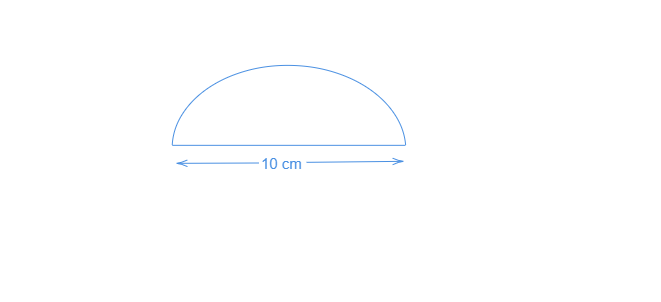# Find the perimeter of the adjoining figure, which is a semicircle including its diameter."

Diameter$=10\ cm$

$r=\frac{10}{2}\ cm$

$=5\ cm$

The perimeter of a semicircle$=\pi r$

$=\frac{110}{7}cm+diameter$

$=\frac{110}{7}\ cm+10\ cm$

$=(\frac{110+70}{7})\ cm$

$=\frac{180}{7}\ cm$

$=25.71\ cm$

Hence the required perometer$=25.7\ cm\ (approx.)$